+
Converting Unit Rates
Solving Linear Equations and Inequalities
0
of 0 possible points

# Converting Unit Rates

Author: Sophia Tutorial
##### Description:

Convert a unit rate in a given scenario.

(more)

Sophia’s self-paced online courses are a great way to save time and money as you earn credits eligible for transfer to many different colleges and universities.*

No credit card required

46 Sophia partners guarantee credit transfer.

299 Institutions have accepted or given pre-approval for credit transfer.

* The American Council on Education's College Credit Recommendation Service (ACE Credit®) has evaluated and recommended college credit for 33 of Sophia’s online courses. Many different colleges and universities consider ACE CREDIT recommendations in determining the applicability to their course and degree programs.

Tutorial
what's covered
1. Converting Units
2. Unit Rates
3. Converting Unit Rates

# 1. Converting Units

When converting from one unit to another, we multiply a quantity by a conversion factor. A conversion factor is a fraction equivalent to 1, so multiplying it by a quantity doesn't change its actual measure. However, the fraction certainly doesn't look like it's equal to one (at least not until you inspect it). The expressions in the numerator and denominator are equal quantities, but measured in different units. During unit conversion, units are also canceled out when they appear in both the numerator and the denominator. To illustrate this idea, let's convert 1.5 feet into inches, using the fact that 1 foot is equivalent to 12 inches.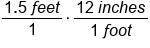Create fraction multiplication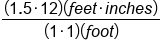Multiply numbers, multiply units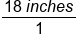Units of feet cancel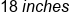Our Solution

# 2. Unit Rates

A unit rate is a ratio between two quantities with different units. For examples, speed is a unit rate, because it is the ratio between distance traveled and the time taken to travel that distance. There are two different types of units that make up this ratio, distance and time. Another characteristic of a unit rate is that a unit rate has a denominator of 1. Let's think about speed again: we wouldn't normally say that a car travels 120 miles per 2 hours. Instead, we simplify the ratio to 1 hour, and say 60 miles per hour.

# 3. Converting Unit Rates

When converting unit rates, we need to make use of multiple conversion factors: as many conversion factors needed to convert from one quantity to another for every unit involved in our unit rate. Let's work more with miles per hour, and convert 60 miles per hour into feet per second. We'll need to convert miles to kilometers, but also hours to seconds. Let's first list our conversion factors: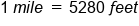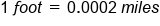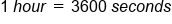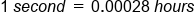hint
When using a conversion factor to create a fraction equal to 1, think about what unit you wish to convert into. In most cases, this will be the numerator of the fraction, which places the unit you wish to cancel in the denominator of that fraction. This is because the unit you wish to cancel will likely be in the numerator of another fraction. Units are canceled when they appear in the numerator and denominator of the combined fraction.
hint
Keep in mind that although we have listed some very helpful conversion factors, we may not need to use all of them. For example, as we will see when we are converting from miles per hour into feet per second, we will use the conversion factor that 1 mile = 5280 feet, instead of 1 foot = 0.0002 miles. This all depends on which unit you want to convert into and which unit you wish to cancel.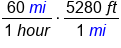This conversion factor cancels miles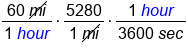The second conversion factor cancels hours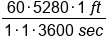Multiply all fractions, noting canceled units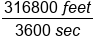Divide 316800 by 3600, for a denominator of 1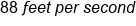Our Solution

summary
When converting units, we multiply a quantity by a conversion factor. Unit rates are ratios comparing two quantities with different units. Conversion factors, which can be used to convert between different units, can also then be used when converting unit rates.

Rating